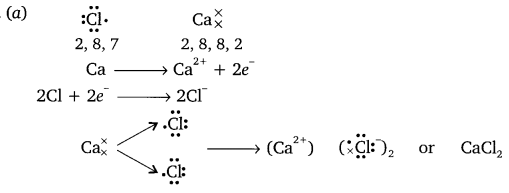# Metals and Non-metals Class 10 Important Questions Science Chapter 3

Solved the very best collection of Metals and Non-metals Class 10 Science Important Questions and Answers Chapter 3 Pdf from the latest NCERT edition books, It will help you in scoring more marks in CBSE Exams.

## Metals and Non-metals Class 10 Important Questions with Answers Science Chapter 3

### Metals and Non-metals Class 10 Important Questions Very Short Answer Type

Question 1.
Name a reducing agent that may be used to obtain manganese from manganese dioxide.   (CBSE 2009)
Aluminium.

Question 2.
A non-metal X exists in two different forms Y and Z. Y is the hardest natural substance, whereas Z is a good conductor of electricity. Identify X, Y and Z.   (CBSE 2011)
‘X’ is carbon, Y is diamond as it is the hardest natural substance and ‘Z’ is graphite as it is a good conductor of electricity.

Question 3.
Why does calcium float on water?   (CBSE 2011)
When calcium is added to water it quickly reacts with it to release hydrogen gas. The bubbles of the hydrogen gas sticks to the surface of calcium and makes it float on water.

Question 4.
Name a non-metal which is lustrous and a metal which is non-lustrous.  (CBSE 2011)
Non-metal: Iodine is lustrous and Metal: Lead is non lustrous.

Question 5.
Which gas is liberated when a metal reacts with an acid? How will you test the presence of this gas?   (CBSE 2011)
Hydrogen gas is formed. Bring a burning matchstick near to it, H2 will burn explosively with ‘pop’ sound.

Question 6.
Name the metal which reacts with a very dilute HNOs to evolve hydrogen gas.   (CBSE 2011)
Magnesium

Question 7.
Name two metals which are found in nature in the free state.   (CBSE 2011)

1. Gold
2. Platinum.

Question 8.
A green layer is gradually formed on a copper plate left exposed to air for a week in a bathroom. What could this green substance be?    (CBSE 2012)
It is due to the formation of basic copper carbonate [CuCO3]

### Metals and Non-metals Class 10 Important Questions Short Answer Type

Question 1.
From amongst the metals sodium, calcium, aluminium, copper and magnesium, name the metal
(i) which reacts with water only on boiling and
(ii) another which doesnot react even with stream.   (CBSE 2008)
(i) Magnesium reacts with water only on boiling
(ii) copper metal doesnot react even with stream

Question 2.
(a) Show the formation of Nacl from sodium and chlorine atoms by the transfer of electrons?
(b) why sodium chloride has a high melting point?
(c) Name the anode and cathode used in electrolytic refining of impure copper metal. (CBSE 2008)(b) Due to strong force of attraction between Na+ and Cl, sodium chloride has high melting point.
(c) Impure copper acts as anode, pure copper acts as cathode.

Question 3.
(a) Show the formation of Na2O by the transfer of electrons between the combining atoms.
(b) Why are ionic compounds usually hared?
(c) How is it that ionic compounds in the solid state do not conduct electricity but they do so when in molton state  (CBSE 2008)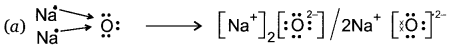(b) Ionic compounds are hard due to strong force of attraction between oppositely charged ions.
(c) In solid state, ions are not free to move where as molton state, ions are free to move, there fore they conduct electricity in molton state.

Question 4.
On adding dilute HCl acid to copper oxide powder, the solution formed is blue-green. Predict the new compound formed which imparts a blue-green colour to the solution.   (CBSE 2008)
The name of the compound is cupric chloride which gives blue-green colour to the solution.
CuO + 2HCl → CuCl2 + H2O

Question 5.
(a) Show on a diagram the transfer of electrons between the atoms in the formation of MgO.
(b) Name the solvent in which ionic compounds are generally soluble.
(c) Why are aqueous solutions of ionic compounds able to conduct electricity? (CBSE 2008)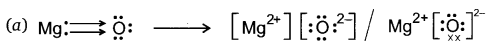(b) Ionic compounds are soluble in water.
(c) The aqueous solutions of ionic compounds consist of ions which can move freely and conduct electricity.

Question 6.
(a) What are amphoteric oxides? Choose the amphoteric oxides from amongst the following oxides?
Na2O, ZnO, Al2O3, CO2, H2O.
(b) Why is it that non-metals do not displace hydrogen from dilute acids?   (AI 2008)
(a) The oxides which are acidic as well as basic in nature are called amphoteric oxides. Al2O3 and ZnO are amphoteric oxides.
(b) Non-metals cannot lose electrons so that H+ ions from acids become hydrogen gas and can be displaced.

Question 7.
Name two metals which react violently with cold water. Write any three observations you would make when such a metal is dropped into water. How would you identify the gas evolved, if any during the reaction?   (AI 2008)
Sodium and potassium react vigorously with cold water.
When these metals are dropped in water, bubbles will be evolved due to evolution of hydrogen gas, large amount of heat is produced and the solution will change red litmus blue.

Hydrogen gas is formed. Bring burning splinter near the mouth of the test tube where gas is evolved, the splinter burns with a pop sound.

Question 8.
(a) Name a metal for each case
(i) it does not react with cold as well as hot water but reacts with steam.
(ii) it does not react with any physical state of water.
(b) When calcium metal is added to water the gas evolved does not catch fire, on adding sodium metal to water catches fire. Why is it so?   (AI 2008)
(a) (i) Iron metal reacts only with steam.
(ii) Copper does not react with any state of water.
(b) Sodium metal is highly reactive. It reacts with water and produces sodium hydroxide and hydrogen gas along with large amount of heat. Due to this large heat, hydrogen catches fire while no such huge amount of heat is released in case of calcium.

Question 9.
Show the electronic transfers in the formation of MgCl2 from its elements.    (Foreign 2008)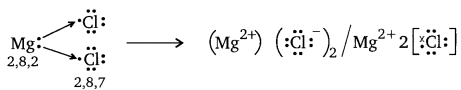Question 10.
Which of the following metals will melt at body temperature (37°C)?    (CBSE 2008C)
gallium, magnesium, caesium, aluminium.
Gallium and caesium.

Question 11.
Give reasons for the following:
(a) Gold and silver are used to make jewellery.
(b) Carbonate and sulphide ores are usually converted into oxides prior to reduction
during the process of extraction.    (CBSE 2008C)
(a) It is because gold and silver are highly lustrous, malleable and ductile.
(b) The reduction of metal oxides into metals is easy, fast and cheap than converting carbonate and sulphide ore into metals.

Question 12.
Give reasons for the following:
(a) Metals can be given different shapes according to our needs.
(b) Hydrogen is not evolved when a metal reacts with nitric acid.
(a) Metals are malleable and ductile i.e. it can be drawn into sheets and wires, it does not break due to high tensile strength and therefore it can be given different shapes according to our needs.
(b) Nitric acid is strong oxidising agent. When metals react with nitric acid it does
not release H2 gas but oxidise it to form H2O.

Question 13.
Give reasons for the following:     (CBSE 2008C, 2012)
(a) Aluminium oxide is considered as an amphoteric oxide.
(b) Ionic compounds conduct electricity in molten state.
(a) Aluminium oxide reacts with acids as well as bases to form salt. It shows the properties of both acid and base.
(b) Ionic compounds produce free ions in molten state which conduct electricity.

Question 14.
The way, metals like sodium, magnesium and iron react with air and water is an indication of their relative positions in the ‘reactivity series’. Is this statement true? Justify your answer with examples.    (CBSE 2011)
The arrangement of metals in the reactivity series is based on the property of their reactivity i.e., how quickly they react in same conditions. The most reactive metal is placed on the top and the least one at the bottom of the series. Sodium will quickly react with water, and magnesium will react slowly and iron metal will take many hours to react. Hence sodium is placed at the top and iron at the bottom based on their ractivity pattern.

Question 15.
X + YSO4 → XSO4 + Y
Y + XSO4 → No reaction
Out of the two elements, ‘X’ and ‘Y, which is more reactive and why?   (CBSE 2011)
‘X’ is more reactive than ‘Y because it displaces ‘Y from its salt solution.

Question 16.
What is an alloy? State the constituents of solder. Which property of solder makes it suitable for welding electrical wires?     (CBSE 2011)
Alloy is a homogeneous mixture of two or more metals. One of them can be a non-metal also. Solder consists of lead and tin. It has low melting point which makes it suitable for welding electrical wires.

Question 17.
Using the electronic configurations, explain how magnesium atom combines with oxygen
atom to form magnesium oxide by transfer of electrons.    (CBSE 2011)Question 18.
When a metal X is treated with cold water, it gives a base Y with molecular formula XOH (Molecular mass = 40) and liberates a gas Z which easily catches fire. Identify X, Y and Z.    (CBSE 2011)
2Na + 2H2O → 2NaOH + H2
Here ‘X’ is Sodium metal, ‘Y is sodium hydroxide and ‘Z’ is Hydrogen gas.

Question 19.
Write chemical equations that shows aluminium oxide reacts with acid as well as base.
(CBSE 2011)
Al2O3 + 6HCl → 2AlCl3 + 3H2O
Al2O3 + 2NaOH → 2NaAlO2 + H2O

Question 20.
Name the following:
(a) A metal, which is preserved in Kerosene.
(b) A lustrous coloured non-metal.
(c) A metal, which can melt while kept on palm.
(d) A metal, which is a poor conductor of heat.    (CBSE 2012)
(a) Sodium is preserved in Kerosine.
(b) Iodine is lustrous non-metal.
(c) Gallium.

Question 21.
Explain the formation of ionic compound CaO with electron number of calcium and oxygen are 20 and 8 respectively.
(b) Name the constituent metals of bronze.    (CBSE 2012)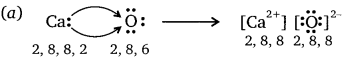(b) Bronze is made up of copper and tin.

Question 22.
A metal ‘X’ acquires a green colour coating on its surface on exposure to air.
(i) Identify the metal ‘X’ and name the process responsible for this change.
(ii) Name and write chemical formula of the green coating formed on the metal.
(iii) List two important methods to prevent the process.     (CBSE 2012)
(i) Metal is copper. The process is corrosion.
(ii) Copper carbonate [CuCO3].
(iii) (a)It should be coated with zinc
(b) It should be mixed with other metals to form alloys.

Question 23.
Write balanced equations for the reaction of:
(i) aluminium when heated in air. Write the name of the product.
(ii) iron with steam. Name the product obtained.
(iii) calcium with water. Why does calcium start floating in water?   (CBSE 2012)
(i) $$4 \mathrm{Al}+3 \mathrm{O}_{2} \stackrel{\text { heat }}{\longrightarrow} 2 \mathrm{Al}_{2} \mathrm{O}_{3}$$
The product formed is aluminium oxide.
(ii) 3Fe + 4H2O (steam) → Fe3O4 + 4H2
The product obtained is iron (II) iron (III) oxide.
(iii) Ca + 2H2O → Ca(OH)2 + H2
The bubbles of hydrogen stick to the surface of metal which when moves up takes metal with it and makes it float.

Question 24.
Write balanced chemical equations for the following reactions:
(a) Dilute sulphuric acid reacts with aluminium powder.
(b) Dilute hydrochloric acid reacts with sodium carbonate.
(c) Carbon dioxide is passed through lime water. (CBSE 2012)
(a) 2Al(s) + 3H2SO4(dil.) → Al2(SO4)3(aq) + 3H2(g)
(b) Na2CO3(s) + 2HCl(dil.) → 2NaCl(aq) + H2O(l) + CO2(g)
(c) Ca(OH)2(aq) + CO2(g) → CaCO3(s) + H2O(l)

Question 25.
(a) Show the formation of Na2O by the transfer of electrons between the combining atoms.
(b) Why are ionic compounds usually hard?
(c) How is it that ionic compounds in the solid state do not conduct electricity but
they do so when in molten state?   (CBSE 2012)(b) It is due to strong force of attraction between oppositely charged ions.
(c) In solid-state, ions are not free to move whereas in molten state ions are free to move, therefore, they conduct electricity in molten state.

Question 26.
(a) Show on a diagram the transfer of electrons between the atoms in the formation of MgO. Write symbols of cation and anion present in MgO.
(b) Name the solvent in which ionic compounds are generally soluble.
(c) Why are aqueous solutions of ionic compounds able to conduct electricity?    (CBSE 2012)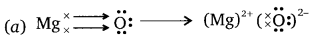MgO contains Mg2+ as cation and O2- as anion.
(b) Ionic compounds are soluble in water.
(c) It is because aqueous solutions consist of ions which can move freely and carry current.

Question 27.
Define the following terms:    (CBSE 2012)
(i) Mineral
(ii) Ore
(iii) Gangue
(i) Mineral: The naturally occurring chemical substance which contains metals with some other elements or impurities is called mineral.
(ii) Ore: The minerals from which metals can be extracted economically on large scale are called ores.
(iii) Gangue: The impurities present in ore/minerals are called gangue.

Question 28.
Give reason for the following:   (CBSE 2013)
(a) School bells are made up of metals.
(b) Electric wires are made up of copper.
(a) It is because metals are sonorous, i.e., they produce sound when struck with a hard substance.
(b) It is because copper is good conductor of electricity and economical.

Question 29.
Suggest a method of reduction for the following metals during their metallurgical processes:   (CBSE 2013)
(i) metal ‘A’ which is one of the last, second or third position in the reactivity.
(ii) metal ‘B’ which gives vigorous reaction even with water and air.
(iii) metal ‘C’ which is kept in the middle of activity series.
(i) A can be obtained by chemical reduction using carbon or carbon monoxide as reducing agent.
(ii) ‘B’ can be obtained by electrolytic reduction.
(iii) ‘C’ can be reduced by reducing agent like Al.

Question 30.
(a) Define activity series of metals. Arrange the metals gold, copper, iron and magnesium in order of their increase in reactivity.   (CBSE 2013)
(b) What will you observe when:
(i) Some zinc pieces are put in copper sulphate solution.
(ii) Some silver pieces are put into green coloured ferrous sulphate solution.
(a) The series of metals in which metals are arranged in decreasing order of their reactivity. Au < Cu < Fe < Mg is increasing order of reactivity.
(b) (i) The blue solution will become colourless and reddish brown copper metal will be deposited.
$$\mathrm{Zn}(\mathrm{s})+\underset{\text { Blue }}{\mathrm{CuSO}_{4}(\mathrm{aq})} \longrightarrow \underset{\text { colourless }}{\mathrm{ZnSO}_{4}(\mathrm{aq})}+\underset{\text { (reddish brown })}{\mathrm{Cu}(\mathrm{s})}$$
(ii) Ag(s) + FeSO4(aq) → No reaction
Reaction will not take place-because ‘Ag’ is less reactive than iron.

Question 31.
You are given samples of three metals. Sodium, magnesium and copper. Suggest any two activities to arrange them in order of decreasing activity.   (CBSE 2014)
Activity: Sodium reacts with cold water vigorously to form sodium hydroxide and hydrogen gas
2Na(s) + 2H2O(l) → 2NaOH(aq) + H2(g)
Magnesium does not react with cold water but with hot water to form magnesium hydroxide and hydrogen gas.
Mg(s) + 2H2O(l) → Mg(OH)2(aq) + H2(g)
Copper does not react even with steam.
Hence sodium is more reactive than magnesium which is more reactive than copper.

Question 32.
Explain why
(a) iron articles are frequently painted.
(b) iron sheets are coated with zinc layer.   (CBSE 2014)
(a) Iron articles when come in contact with oxygen and water (moisture), rust to form flaky substance on its surface and slowly loses its strength. To avoid this it is painted frequently.
(b) Iron sheets are coated with zinc (galvanisation) so as to prevent iron from rusting.

Question 33.
Write one example of each of
(i) a metal which is so soft that, it can be cut with knife and a non-metal which is the hardest sustance.
(ii) a metal and a non-metal which exist as liquid at room temperature.    (CBSE 2015)
(i) Sodium, carbon (diamond).
(ii) Mercury is liquid metal, bromine is liquid non-metal.

Question 34.
Mention the names of the metals for the following:    (CBSE 2015)
(i) Two metals which are alloyed with iron to make stainless steel.
(ii) Two metals which are used to make jewellary.
(i) Nickel and chromium.
(ii) Gold and platinum.

Question 35.
State three reasons for the following facts
(i) Sulphur is a non-metal
(ii) Magnesium is a metal
One of the reasons must be supported with a chemical equation.   (CBSE 2015)Question 36.
What is cinnabar? How is metal extracted from cinnabar? Explain briefly.   (CBSE 2015)
Cinnabar is HgS.
Mercury is obtained by roasting cinnabar. HgO formed is thermally unstable and gives mercury.Mercury can purified by distillation.

Question 37.
(a) Write the electron dot structures for potassium and chlorine.
(b) Show the formation of KCl by the transfer of electrons.
(c) Name the ions present in the compound KCl.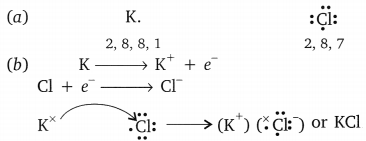(c) KCl has K+ and Cl.

Question 38.
(a) State the electron-dot structures for calcium and sulphur.
(b) Show the formation of CaS by the transfer of electrons.
(c) Name the ions present in this compound CaS. Atomic number of Ca = 20, O = 16.   (CBSE 2015)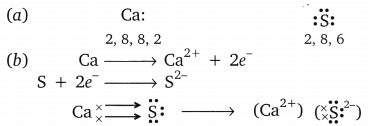(c) Ca2+ and S2- ions are present in CaS.

### Metals and Non-metals Class 10 Important Questions Long Answer Type

Question 1.
Sample pieces of 5 metals A, B, C, D and E are added to the tabulated solutions separately. The results observed are shown in the table.   (Foreign 2008)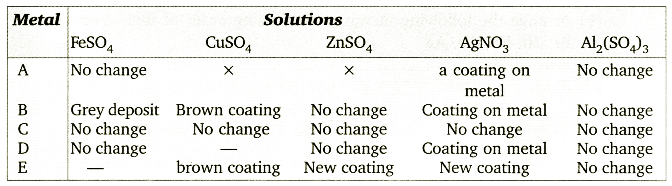Based on the observations recorded in the table, answer the following?
(i) Which is the most reactive metal?
(ii) Which is the least reactive metal?
(iii) What would be observed if metal D were added to a solution of copper (II) sulphate?
(iv) What would be observed if metal E were added to a solution of iron (II) sulphate?
(v) Arrange the metals A, B, C, D and E in order of decreasing reactivity.
(i) The most reactive metal is E as it displaces Zn from ZnSO4 solution.
(ii) The least reactive metal is C as it cannot displace even Ag from AgNO3.
(iii) If ‘D’ is more reactive than copper, then the brown coating on the metal would be formed.
(iv) ‘E’ is more reactive than Fe, hence it can displace Fe form FeSO4, a grey coating on metal will be formed.
(v) E > B > D > A > C

Question 2.
(a) With a labelled diagram, describe an activity to show that metals are good conductors of electricity.
(b) Account for the following:
(i) Hydrogen gas is not evolved when a metal reacts with nitric acid.
(ii) For storing, sodium metal is kept immersed in kerosene.
(iii) The reaction of iron (III) oxide, Fe2O3 with aluminium is used to join cracked iron parts of machines. (Foreign 2008)
(a) Activity: To show that metals are good conductors of electricity.
Materials: Metal pieces, like Zn, Fe, Cu and Al, connecting clips, battery, bulb, and wires.
Set the apparatus as shown in the figure, place different metals between the clips A and B. The bulb glows when all of these metals are placed between the clips.
This proves that metals are good conductors of electricity.(b) (i) Nitric acid is a strong oxidising agent, it oxidises the H2 gas to H2O.
(ii) Sodium is very reactive metal, it reacts with water and oxygen at room temperature, but it does not react with kerosene, so it is stored under kerosene.
(iii) The reaction of aluminium with iron (III) oxide is highly exothermic, it produces iron metal in molten form, which helps in joining the cracked iron parts of a machine.

Question 3.
(a) Describe an activity to find out which metal is more reactive-iron or copper?
(b) Arrange the following metals in decreasing order of their reactivity.
Fe, Zn, Na, Cu, Ag
(c) Why do ionic compounds have high melting points?
(d) Show the formation of NaCl from Na and Cl atoms by the transfer of electrons.   (AI 2008C)
(a) To find more reactive metal among iron and copper.
Arrange the following setup:In test tube A, iron metal when dipped in CuSO4 it displaces copper from its solution to form iron sulphate and copper metal gets deposited on the surface of iron metal.
In test tube B – No reaction takes place. This shows that iron is more reactive than copper.
(b) Na > Zn > Fe > Cu > Ag
(c) Ionic compounds have high melting point because there are strong forces of attraction between ions due to which large amount of energy is required to break this bond.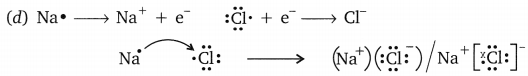Question 4.
(a) Give an example of a metal which
(i) can be easily cut with a knife,
(ii) is a liquid at room temperature.
(b) Write chemical equation for the reaction when
(i) Steam acts on red hot iron.
(ii) Zinc is added to iron (II) sulphate solution.
(c) What are alloys?
(d) Why are food cans coated with tin and not zinc?   (AI 2008C)
(a) (i) Sodium can be cut with knife.
(ii) Mercury is liquid at room temperature.
(b) (i) 3Fe(s) + 4H2O(g) → Fe3O4(s) + 4H2(g)
(ii) Zn(s) + FeSO4(aq) → ZnSO4(aq) + Fe(s)
(c) Alloys are homogeneous mixture of two or more than two metals or metals and non-metals.
(d) Food cans are coated with tin and not zinc. Because tin is less reactive than zinc it does not corrode easily and it is cheaper.

Question 5.
(a) Name the main ore of mercury. How is mercury obtained from its ore? Give balanced chemical equation.
(b) What is thermite reaction? How is it used to join the railway tracks or craked machine parts?
(c) Name the method used to extract metals of high reactivity.   (CBSE 2011)
(a) Cinnabar
Mercury is obtained from its ore by roasting.
HgS + O2 → Hg + SO2
(b) When aluminium is heated with Fe2O3 to get molton iron, it is called thermite reaction.
Fe2O3 + 2Al → Al2O3 + 2Fe
Molten iron is used to weld broken railway tracks.
(c) Electrolytic reduction.

Question 6.
(a) How can the metals at the top of the reactivity series be extracted from their ores? Explain with an example.
(b) Name any one alloy made from
(i) a metal and a non-metal, and
(ii) two metals.      (CBSE 2011)
(a) These metals are extracted by electrolytic reduction, e.g., aluminium is obtained
from bauxite by electrolytic reduction.
(b) (i) Steel is made up of iron and carbon.
(ii) Brass is made up of copper and zinc.

Question 7.
(a) Give two methods to prevent the rusting of iron.
(b) Name the ores of the following metals:
(i) mercury, and
(ii) zinc
(c) Explain with the help of a diagram, how copper metal can be refined? Label the
important arrangements in the experimental setup.   (CBSE 2011)
(a) (i) Painting
(ii) Galvanisation
(b) (i) Cinnabar
(ii) Zinc Blende
(c) Impure copper is taken as anode whereas , pure copper is taken as cathode. Copper sulphate solution (CuS04) is taken as electrolyte. When electric current is passed, impure copper changes to ions which gain electrons at cathode and change into pure copper. Impurities are left behind as anode mud.
At anode: Cu(s) → Cu2+(aq) + 2e
At cathode: Cu2+(aq) + 2e → Cu(s)Question 8.
With the help of a suitable example, explain how ionic compounds are formed. State any three general properties of ionic compounds. (CBSE 2011)
Ans. Ionic compounds are formed by transfer of electrons from metal to non-metals, e.g.General Properties:
(i) They are the solids having high melting point.
(ii) They are soluble in water.
(iii) They conduct electricity in molten state as well as in aqueous solution.

Question 9.
(a) Explain with an example how the metal (X) which is low in reactivity series and metal (Y) which is high in the reactivity series are obtained from their compounds by reduction process.
(b) Write the electronic configuration of sodium and chlorine. Show the formation of sodium chloride from sodium and chlorine by the transfer of electrons.
(c) List any two observations when a highly reactive metal is dropped in water.   (CBSE 2011)
(a) ‘X’ is obtained by chemical reduction with the metals high in reactivity series. ‘Y is obtained by electrolytic reduction.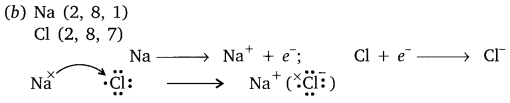(c) (i) Metal will catch fire.
(ii) Alkali solution is formed which turns red litmus blue.

Question 10.
The reaction of metal (X) with ferric oxide is highly exothermic. Metal (X) is obtained from its oxides by electrolytic reduction. Identify (X) and write its reaction with ferric oxide.
(b) Give reason to justify that aluminium oxide is an amphoteric oxide. Also, give another example of amphoteric oxide.
(c) Mention constituent metals present in bronze.   (CBSE 2011)
(a) ‘X’ is ‘Al’
2Al(s) + Fe2O3(s) → Al2O3(s) + 2Fe(l) + Heat
(b) Al2O3 reacts with acid as well as base, therefore it is amphoteric oxide.
Al2O3 + 2NaOH → 2NaAlO3 + H2O
Al2O3 + 6HCl → 2AlCl3 + 3H2
Zinc oxide is also an amphoteric oxide.
(c) Bronze contains copper and tin.

Question 11.
(a) Write the chemical name of the coating that forms on silver and copper articles when these are left exposed to moist air.
(b) Explain what is galvanisation. What purpose is served by it?
(c) Define an alloy. How are alloys prepared? How do the properties of iron change when:
(i) small quantity of carbon,
(ii) nickel and chromium are mixed with it.    (CBSE 2012)
(a) On silver, silver sulphide layer is formed and on copper, copper carbonate layer is formed.
(b) The coating of zinc metal over the surface of iron metal is called galvanization. It is done to prevent the rusting of iron.
(c) Alloy is a homogeneous mixture of two or more metals or metal and non-metals. They are prepared by melting the two components of alloy metals together.
(i) On adding small quantity of carbon in iron, steel is obtained which does not rust.
(ii) iron, when mixed with nickel and chromium, forms an alloy named stainless steel which also does not rust.

Question 12.
(a) Differentiate between roasting and calcination. Explain the two with the help of suitable chemical equations. How is zinc extracted from its ore?
(b) Name two metals that can be used to reduce metal oxides to metals.   (CBSE 2012)
(a) Roasting: It is a process in which sulphide ore is heated in the presence of oxygen to convert into oxide.
2Zns + 3O2 → 2ZnO + 2SO2
Calcination: It is a process in which carbonate ore is heated in the absence of air to form oxide.
ZnCO3(s) → ZnO(s) + CO2(g)
After calcination, Zn can be extracted by reduction with carbon.
Reduction: ZnO + C → Zn + CO
(b) Aluminium, Magnesium.

Question 13.
(a) In the formation of compound between two atoms A and B, A loses two electrons and B gains one electron.
(i) What is the nature of bond between A and B?
(ii) Suggest the formula of the compound formed between A and B.
(b) On similar lines explain the formation of MgCl2 molecule.
(c) Common salt conducts electricity only in the molton state. why?
(d) Why is melting point of NaCl high?     (CBSE 2012)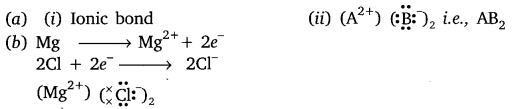(c) Na+ and Cl are free to move in molten state but not in solid state.
(d) It is due to strong force of attraction between Na+ and Cl.

Question 14.
(a) Carbon cannot be used as reducing agent to obtain Mg from MgO. Why?
(b) How is sodium obtained from molten sodium chloride? Give equation of the reactions.
(c) How is copper obtained from its sulphide ore? Give equations of the reactions.   (CBSE 2012)
(a) It is because ‘Mg’ is more reactive or stronger reducing agent than carbon.
(b) Sodium is obtained from molten NaCl by electrolysis.
$$\underset{\text { (Molten })}{2 \mathrm{NaCl}} \stackrel{\text { electrolysis }}{ } \rightarrow 2 \mathrm{Na}(\mathrm{s})+\mathrm{Cl}_{2}(\mathrm{g})$$
At anode: 2Cl → Cl2(g) + 2e
At cathode: 2Na+ 2e → 2Na(s)
(c) Copper ore is concentrated by froth-floatation process.
Roasting: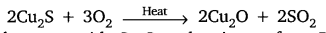Copper oxide reacts with Cu2S on heating to form Blister copper and SO2.Question 15.
How is the method of extraction of metals high up in the reactivity series different from that for metals in the middle? Why the same process cannot be applied for them? Explain giving equations, the extraction of sodium. (CBSE 2012)
Metals high up in the series are obtained by electrolytic reduction because these metals are strong reducing agents and therefore, cannot be obtained by chemical reduction.

Metals in middle of series are less reactive and can be obtained by chemical reduction with the metals that lie above in the series. The same process cannot be used for both of them as highly reactive metals cannot be obtained by chemical reduction. Extraction of sodium is done by electrolysis of molten sodium chloride.Question 16.
Write the names and symbols of two most reactive metals. Explain by drawing electronic structure how any one of the two metals react with a halogen. State any four physical properties of the compound formed.    (CBSE 2012)
K(Potassium) and Na(Sodium) are the two most reactive metals.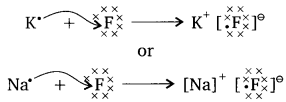where ‘F’ is a halogen.
Four physical properties of the compounds formed by these elements and halogens are:
(i) They have high melting point.
(ii) They are soluble in water.
(iii) They conduct electricity in molten state, not in solid state.
(iv) They are solid and somewhat hard.

Question 17.
Give reasons for the following:
(i) Silver and copper lose their shine when they are exposed to air. Name the substance formed on their surface in each case.
(ii) Tarnished copper vessels are cleaned with tamarind juice.
(iii) Aluminium is more reactive than iron, yet there is less corrosion of aluminium as compared to iron when both are exposed to air.    (CBSE 2012)
(i) These metals get corroded. Silver forms black Ag2S (silver sulphide) and copper form greenish layer of CuCO3 (copper carbonate).
(ii) Tamarind contains acid which reacts with basic copper carbonate and product gets dissolved and removed from copper vessel.
(iii) Aluminium forms oxide layer on its surface which does not further react with air.

Question 18.
(a) Write the electron dot structures of sodium, oxygen and magnesium.
(b) Show the formation of Na2O and MgO by transfer of electrons. Name the ions present in these compound.
(c) List three properties of ionic compounds.    (CBSE 2012)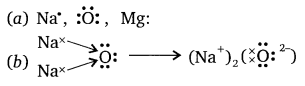Na2O contains Na+ and O2- ions.
MgO contains Mg2+ and O2- ions.

(c) (i) They are solids having high melting point.
(ii) They are soluble in water.
(in) They conduct electricity in molten state as well as in aqueous solution.

Question 19.
What are alloys? How are they made? Name the constituents and uses of brass, bronze and solder.    (CBSE 2012)
Alloys are homogenous mixtures of two of more metals. One of them can be a non-metal. They are made by melting a metal which is in large amount and then add the other metal in molten form. Brass contains copper and zinc. It is used for making decorative articles. Bronze contains copper and tin. It is used for making statues and medals. Solder contains lead and tin. It is used for soldering purposes.

Question 20.
A metal (E) is stored under kerosene. When a small piece of it is left open in the air, it catches fire. When the product formed is dissolved in water, it turns red litmus to blue.
(i) Name the metal (E).
(ii) Write the chemical equation for the reaction when it is exposed to air and when the product is dissolved in water.
(iii) Explain the process by which the metal is obtained from its molten chloride.     (CBSE 2012)
(i) Metal E is sodium
(ii) Reaction of sodium metal with air is:
4Na + O2 → 2Na2O
When the product formed is dissolved in water:
Na2O + H2O → 2NaOH
(iii) The molten chloride of sodium metal is NaCl, the metal can be obtained by the electrolytic reduction. The current is allowed to pass through the molten sodium chloride using the two carbon electrodes. The sodium metal is obtained at the cathode and the chlorine gas is collected at the anode.
2NaCl → 2Na + Cl2

Question 21.
Write two differences between calcination and roasting.   (CBSE 2012)

Question 22.
You are provided with magnesium ribbon and sulphur powder. Explain with the help of an activity that metal oxides are basic and non-metal oxides are acidic in nature.    (CBSE 2014)
Activity:

• Take magnesium ribbon with a pair of tongs and bum it in flame in the presence of air.
• Collect the product formed and dissolve it in warm water.
• Add red litmus paper into it.
• Observe the change in colour and decide the nature of the oxide formed.
• Bum sulphur in a deflagrating spoon in the presence of air, collect the gas in an inverted test tube and dissolve the oxide formed in water.
• Dip blue litmus paper into the solution and observe the change in the colour and decide the nature of the oxide formed.

Observation:
The oxide formed by magnesium metal turns red litmus blue whereas oxide of sulphur, a non-metal turns blue litmus red.
Chemical Reactions:Conclusion: Most of the metallic oxides are basic in nature whereas most of the non- metallic oxides are acidic.

Question 23.
(a) An ore on treatment with dilute hydrochloric acid produces brisk effervesces.
What type of ore is this? What steps will be required to obtain metal from the enriched ore.
(b) Copper coin is kept immersed in silver nitrate solution for some time. What change will take place in coin and colour of the solution? Write balanced chemical equation of the reaction involved.    (CBSE 2014)
(a) Carbonate ore:
(i) Calcination: Carbonate ore is heated in limited supply of air and oxide is obtained, e.g.
ZnCO3(s) → ZnO(s) + CO2(g)
(ii) Reduction with carbon: Oxide ore is then heated with carbon to obtain metal.
ZnO(s) + C(s) → Zn(s) + CO(g)
(b) Copper, being more reactive than silver, will displace silver from silver nitrate solution and there will be deposition of silver on copper coin. The colour of solution will turn to blue.
Cu(s) + 2AgNO3(aq) → Cu(NO3)2(aq) + 2Ag(s)

Question 24.
(a) Write electron dot diagram for chlorine (at no. 17) and calcium (at no. 20). Show the formation of calcium chloride by transfer of electrons.
(b) Identify the nature of above compound and explain three physical properties of such compound.   (CBSE 2015)